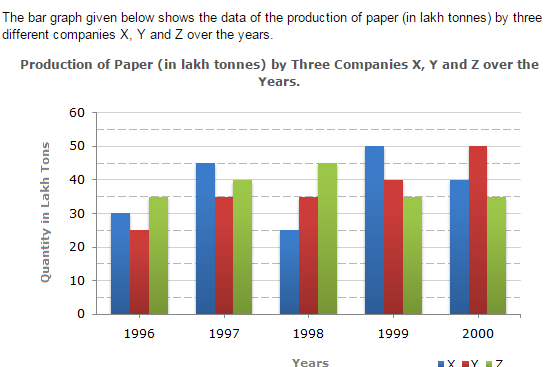Direction1.

For which of the following years, the percentage rise/fall in production from the previous year is the maximum for Company Y?

 A. 1997 B. 1998 C. 1999 D. 2000
Explanation: Percengate change (rise/fall) in the production of Company Y in comparison to the previous year, for different years are: For 1997 = [ (35 - 25) x 100 ] % = 40%. 25 For 1998 = [ (35 - 35) x 100 ] % = 0%. 25 For 1999 = [ (40 - 35) x 100 ] % = 14.29%. 35 For 2000 = [ (50 - 40) x 100 ] % = 25%. 40 Hence, the maximum percentage rise/fall in the production of Company Y is for 1997.

2.

What is the ratio of the average production of Company X in the period 1998-2000 to the average production of Company Y in the same period?

 A. 1:1 B. 15:17 C. 23:25 D. 27:29
Explanation: Average production of Company X in the period 1998-2000 = [ 1 x (25 + 50 + 40) ] = ( 115 ) lakh tons. 3 3 Average production of Company Y in the period 1998-2000 = [ 1 x (35 + 40 + 50) ] = ( 125 ) lakh tons. 3 3 Therefore Required ratio = ( 115 ) 3 = 115 = 23 . ( 125 ) 3 125 25

3.

The average production for five years was maximum for which company?

 A. X B. Y C. Z D. X and Z both
Explanation: Average production (in lakh tons) in five years for the three companies are: For Company X = [ 1 x (30 + 45 + 25 + 50 + 40) ] = 190 = 38. 5 5 For Company Y = [ 1 x (25 + 35 + 35 + 40 + 50) ] = 185 = 37. 5 5 For Company Z = [ 1 x (35 + 40 + 45 + 35 + 35) ] = 190 = 38. 5 5 Therefore Average production of five years is maximum for both the Companies X and Z.

4.

In which year was the percentage of production of Company Z to the production of Company Y the maximum?

 A. 1996 B. 1997 C. 1998 D. 1999